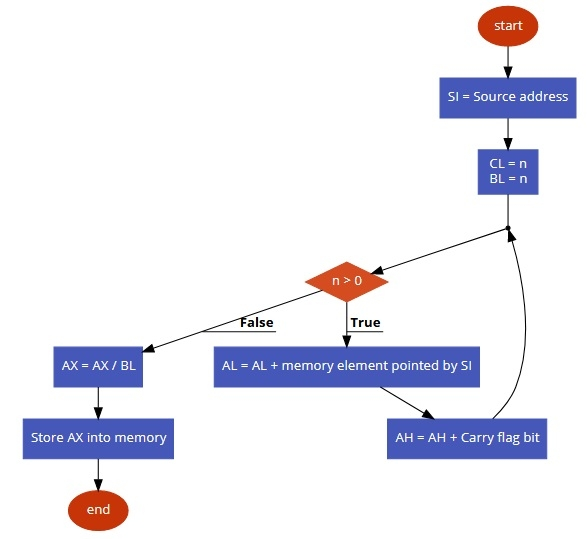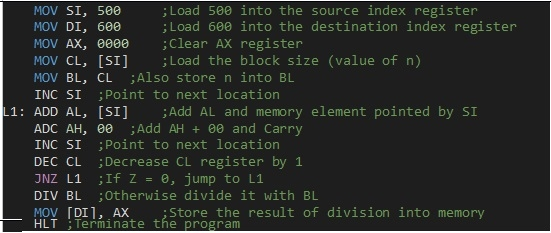# 8086 program to find average of n numbers

In this program we will see how to find the average of n numbers in a given series.

## Problem Statement

Write 8086 Assembly language program to find the average of n numbers stored in a given series starts from memory offset 501. The size of the series is stored at memory offset 500.

## Discussion

To do this task we are initializing the Source Index (SI) register to the starting address of the series. We are also taking the series size into CL. The CL will be used as counter. To store add we are using AL register. Initially set AL to 0. In each iteration we are taking the number from the memory and add them one by one. After addition we are dividing the number with the size of the series to get the average.

Here we are taking the numbers (CF, 24, 2C, 51, 3E. 45) = 1F3. And average is 53

Data

500
06
501
CF
502
24
503
2C
504
51
505
3E
506
45

## Flow Diagram## Program